# Astro 1102/1104: Section 2¶

In :
from IPython.display import Latex
import matplotlib.pyplot as plt
%matplotlib inline
import numpy
from matplotlib import animation, rc
from IPython.core.display import HTML
from IPython.display import HTML
from ipywidgets import *
from scipy.integrate import odeint
from IPython.display import Image


## Seasons¶

Each planet is doing two things: it is orbiting the Sun and it is spinning. The path that the planet takes about the Sun lies entirely in a plane (we could take a huge, flat piece of paper and, oriented properly, the planet would stay on the surface of that piece of paper). The axis about which the planet spins does not necessarily point directly off of the plane.

Let's assume for the moment that a planet is in a circular orbit (eccentricity is zero, foci on top of one another), and that the spin axis points directly out of the plane of orbit.

In :
PATH = "/Users/hunteradams/Documents/PhD Semester 5/Sections/"
Image(filename = PATH + "flat.png", width=600, height=600)

Out: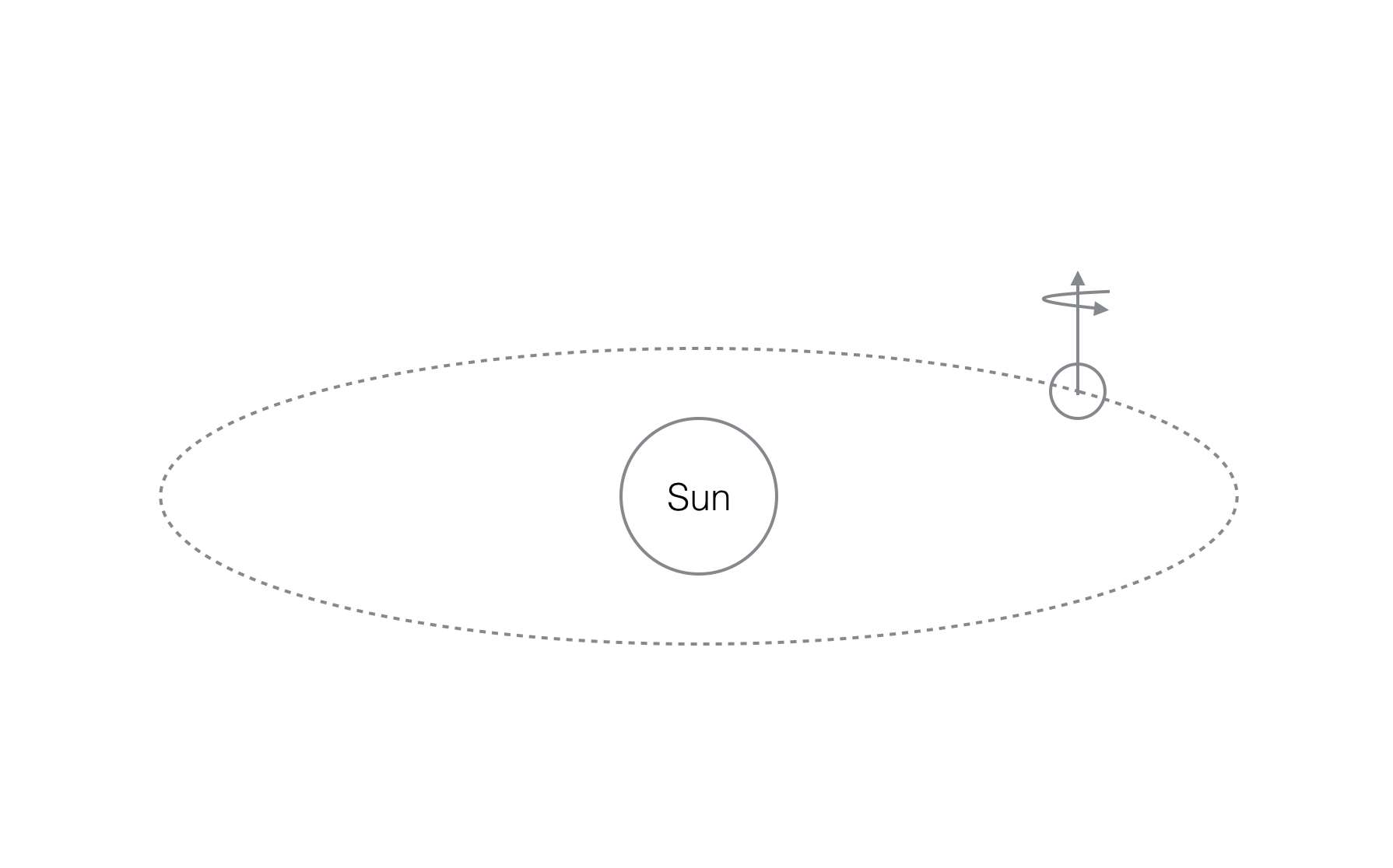Question: How does the length of a day change throughout the year for this planet?

Answer: It doesn't! Why? No matter where the planet is in its orbit, the Sun traverses the same path in the sky everyday. Remember that half of the Earth is always illuminated. So if the axis of rotation is not tilted, then for any day of the year the size of the illuminated part of the sphere that I spin through stays the same.

Question: Is there a particular time of the year that would be hotter/colder for any part of this planet than any other time?

Answer: No! For any latitude, the sunlight intensity is always the same. Thus, there are no seasons.

Now let's assume that the spin axis is tilted relative to the orbital plane (i.e. the planet has some obliquity). How do the answers to the above questions change?

In :
Image(filename = PATH + "tilt.png", width=600, height=600)

Out: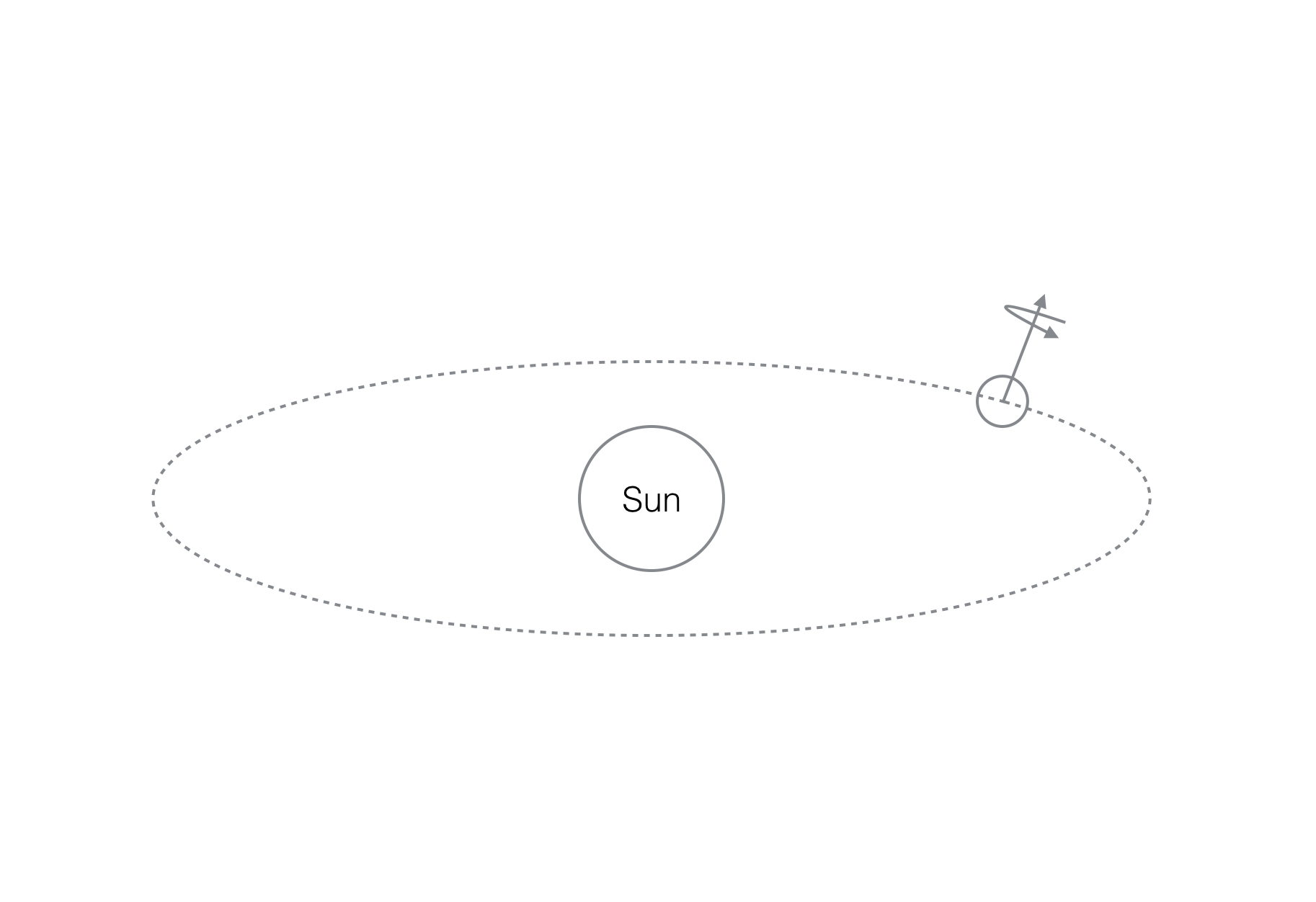Answer: Depending which side of the Sun I'm on (and which part of the planet), the day is longer or shorter.

Answer: If I'm tilted toward the Sun, my days are longer and my sunlight is more direct (the sun is higher in my sky). So, part of the year will have longer, hotter days; and the other part will have shorter, colder days.

A sidenote. Eccentricity can also affect this. What if the Earth's orbit were highly eccentric? We would then have seasons of different duration (Kepler's second law).

Question: Why does the sun never set during an Arctic or Antarctic summer?

## Sidereal vs. Solar Days¶

Let's consider just the spinning Earth (forget about the Sun for a moment). When we're talking about sidereal time, we're considering the planet as an individual entity. If we're looking straight down on the North Pole, we'll see the Earth spinning counterclockwise. The sidereal day is the amount of time it takes for the Earth to complete one rotation ($2 \pi$ radians, 360 degrees).

In :
Image(filename = PATH + "earthspin.png", width=600, height=600)

Out: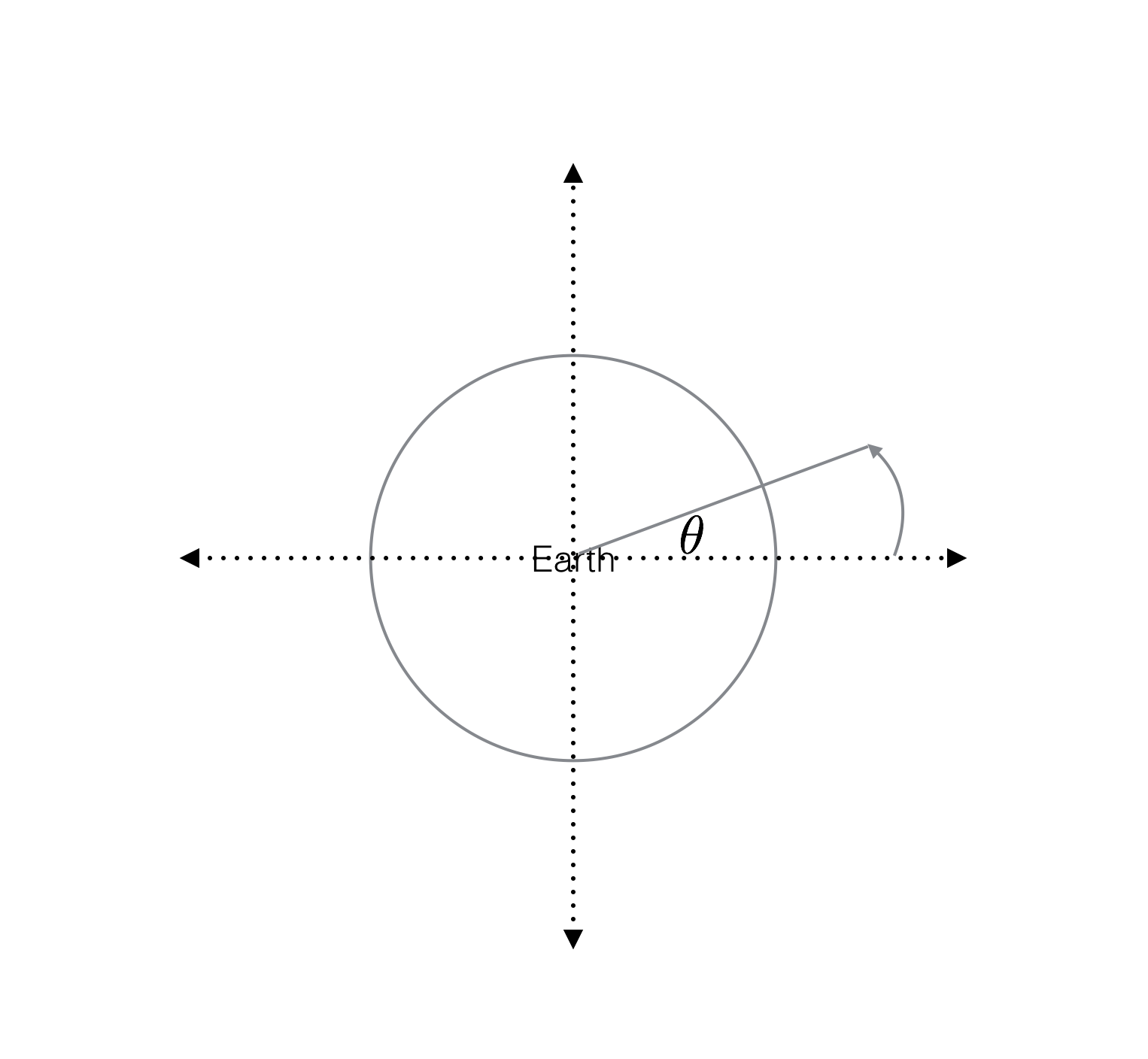Now let's bring the Sun into the picture. Suppose that instead of asking how long it takes for the Earth to spin 360 degrees, we instead asked how long it takes for the Sun to return to the same spot in the sky. If we're spinning in the same direction that we're orbiting, it's going to take longer than a sidereal day because the Earth will have moved relative to the Sun. What if we're spinning opposite the direction that we're rotating?

In :
Image(filename = PATH + "earthsun.png", width=600, height=600)

Out: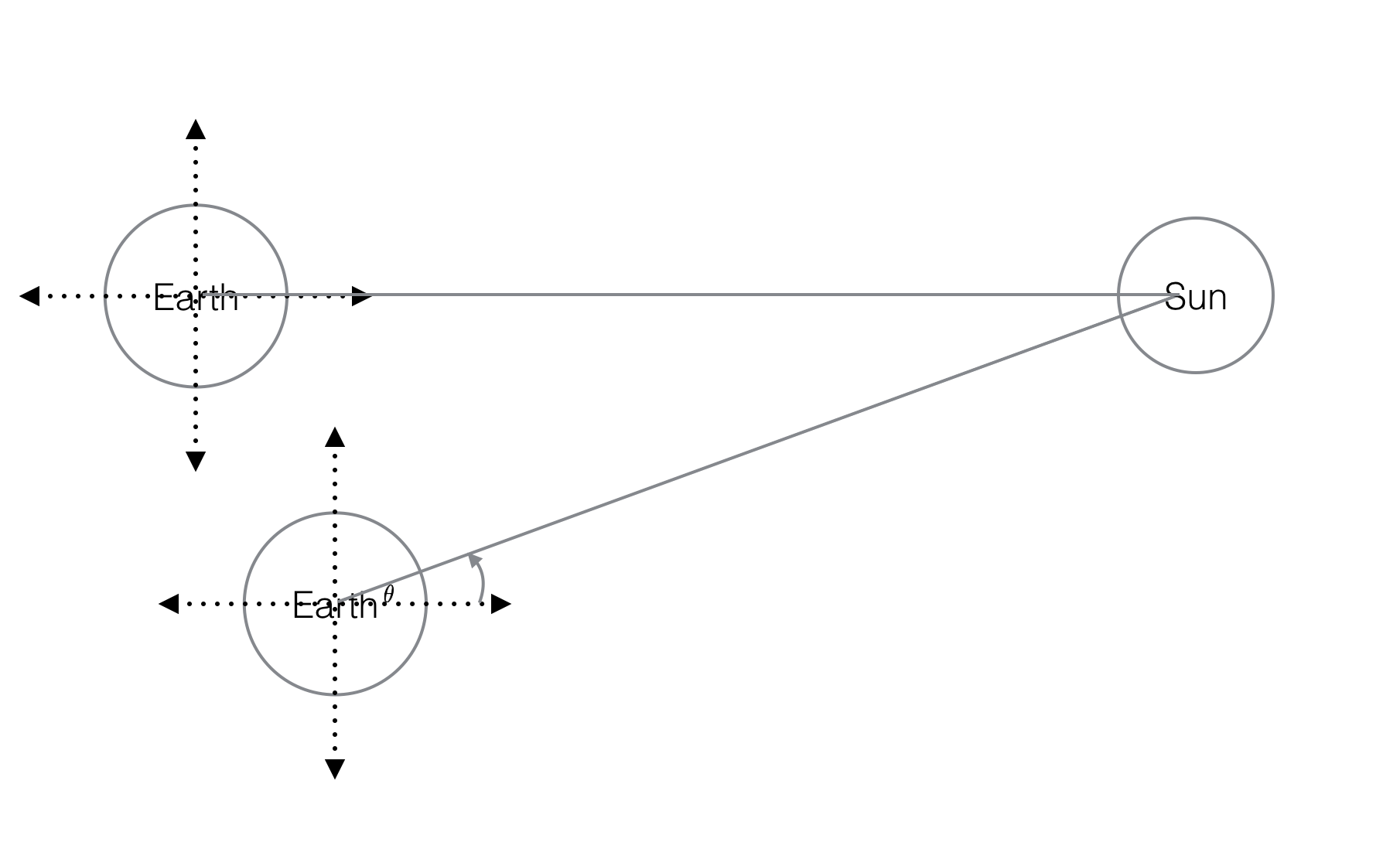## Moon Phases and Geometry¶

When you look at the Moon in the sky, you gather two pieces of information:

1. The phase of the Moon
2. The location of the Moon in the sky (South, North, East, West)

These two pieces of information can be used to tell approximately what time it is. The first piece of information (the phase of the Moon) defines the geometry of the Earth-Sun-Moon system. The second piece of information (location in sky) defines the time of the day/night. To understand this, we can draw a picture:

In :
Image(filename = PATH + "geometry.png", width=600, height=600)

Out: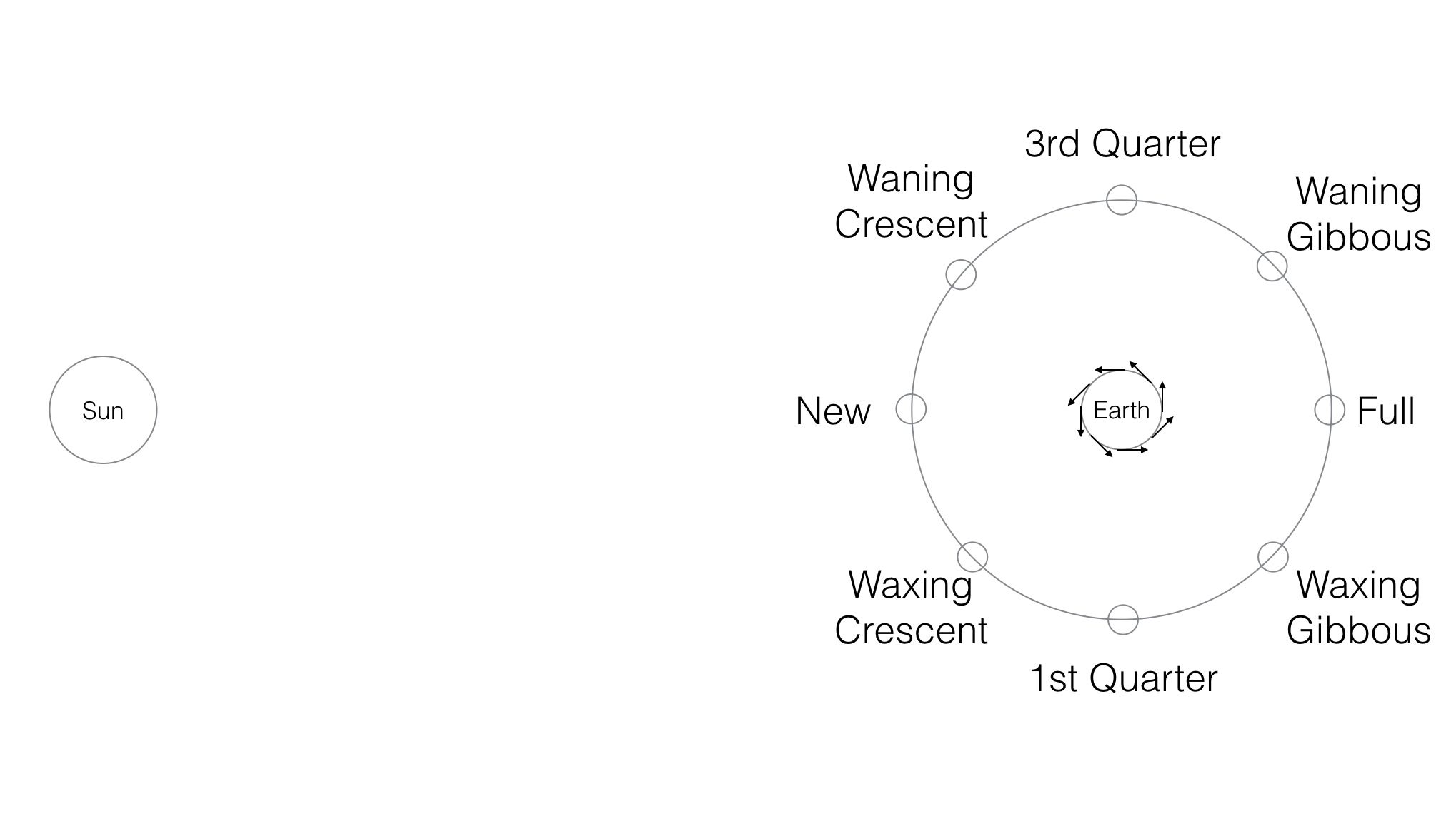Consider this example:

In :
Image(filename = PATH + "example.png", width=600, height=600)

Out: